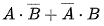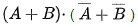# XOR Gate | Symbol, Truth table & Circuit

A XOR gate is a gate that gives a true (1 or HIGH) output when the number of true inputs is odd. An XOR gate is also called exclusive OR gate or EXOR. In a two input XOR gate, the output is high or true when two inputs are different.

In Boolean expression, the term XOR is represented by symbol (⊕) and the Boolean expression is represented as Y = A ⊕ B. It is read as “A xor B”.

## XOR gate symbol

The logic symbol of XOR gate is shown in figure 1.

## XOR gate truth table

The XOR gate truth table for figure 1 is shown below.

 A B Y = A ⊕ B 0 0 0 0 1 1 1 0 1 1 1 0

From XOR gate truth table, it can be concluded that the output will be logical 1 or high when number of true inputs is odd.

Y = A ⊕ B

Y ==Above algebraic expression represent the XOR gate with inputs A and B.

Note:### XOR gate using OR, AND, and NOT gate

To produce XOR gate using OR, AND, and NOT gate, the gates are joined together as shown in figure 2.

### XOR gate using NOR gate

To produce XOR gate using 4 NOR gates, the NOR gates are connected as shown in fig. 3.

To produce XOR gate using 5 NOR gates, the NOR gates are connected as shown in fig. 3.